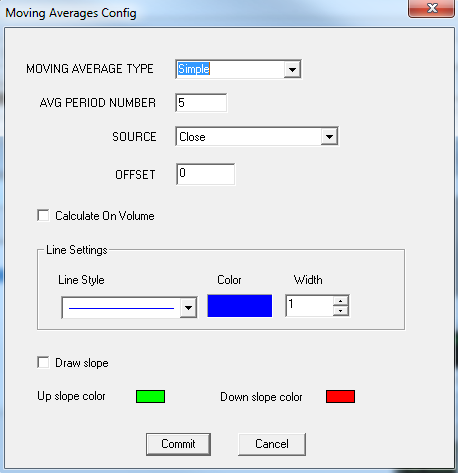# Moving Average

Moving Average study allows user add a customized moving average line to their chart. User can add this study to their chart by right click the chart and select Study Config to open the study configure window first, then select the “Moving Average” from the study list and add it into the Studies In Chart field.Moving Average Configure window allows user to modify the moving average chart, such as change the moving average types and line colors. User can open this Moving Average Configure window either by click the “Config” button or double click the study “Moving Average” under Studies In Chart section.

## Explanation of Each Field

·         Moving Average Type: User can use the dropdown menu to change the moving average type:

Ø  Simple: Selected this to use the simple moving average.

Ø  Weighted: Selected this to use the weighted moving average.

Ø  Exponential: Selected this to use the exponential moving average.

Ø  Smooth: Selected this to use the smooth moving average.

Ø  Volume Weighted: Selected this to use the volume weighted moving average.

·         AVG Period Number: Enter the study period for the selected moving average.

·         Source: Use the dropdown menu to select the study source for the selected moving average study.

Ø  Open: Use the open price data to calculate the moving average.

Ø  High: Use the highest price data to calculate the moving average.

Ø  Low: Use the lowest price data to calculate the moving average.

Ø  Close: Use the close price data to calculate the moving average.

Ø  (High+Low)/2: Use this equation to calculate the price first, then use those price data to calculate the moving average.

Ø  (High+Low+Close)/3: Use this equation to calculate the price first, then use those price data to calculate the moving average.

Ø  (Open+High+Low+Close)/4: Use this equation to calculate the price first, then use those price data to calculate the moving average.

(Hint, those Open, High, Low, and close are represents each bar’s open price, highest price, lowest price, and close price.)

·         Offset: Allows user to view the offset chart.

·         Calculate On Volume: Allows user to calculate the moving average base on the volume instead of price.

·         Line Settings: Allows user to modify the moving average line.

Ø  Line Style: Allows user to use the dropdown menu to change the line style.

Ø  Color: Click the color field allows user to change the line color.

Ø  Width: Allows user to adjust the line’s width.

·         Draw Slope: Allows user to add slopes to the chart. And user can click the color fields to change the color for up slope and down slope.